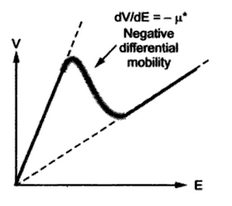### Gunn Diodes

The Gunn diode is discovered by J.B. Gunn. The Gunn diodes are microwave devices which use the transferred electron mechanism, which is also called Gunn effect. The normal diodes have p-n junction but there is no p-n junction in Gunn diodes. These devices are called diodes they have two terminals. The operation of the Gunn diode is dependent on a semiconductor material having different valleys in conduction band at different energy levels. The gallium arsenide (GaAs) satifies this requirement. Let us study the transferred electron mechanism, which is the principle of working of Gunn diode.
1. Transferred Electron Mechanism
Consider the energy band diagram of GaAs as shown in the Fig. 1.Fig.1 energy band diagram of GaAs
The conduction band has different valleys. Under normal conditions the electrons are in a valley which is called central valley. The GaAs is direct band gap material where minimum  of central valley corresponds to the maximum of valence band. The central valley is at lowest energy level. The other valleys are called satellite valleys which are at higher energy levels compared to central valley. The Fig. 1 shows a satellite valley which is 0.3 eV higher than the energy level of central valley.
It is known that the velocity of electrons is related to an applied electric field by the relation,
V = µn E
Where µn = Mobility of electrons
The mobility is constant over a range of applied electric field E. thus under normal operation, as electric field E increases, the velocity of electrons increases. But this happens till E reaches upto a critical value which is about 3 kV/cm. Above this value, mobility µn starts behaving nonlinearily.
When electric field increases beyond the critical value, the electrons in central valley gain enough energy to transfer from central valley to the valley at higher energy level. This is shown in the Fig. 2. The electrons remain in the satellite valley till the field is greater than the critical value.Fig. 2 Electron transfer
Practically there are number of satellite valleys at same energy level exist in number of directions. For simplicity only one such valley is shown in the Fig. 2. Such a transfer of electrons under extremely high field, from central valley to other satellite valleys is called transferred electron mechanism.
Effect of transferred electron mechanism : The electrons located in satellite valleys show the properties related to energy level of that region of conduction band. The effective mass of such electrons is higher than the effective mass of electrons in central valley. It is almost eight time higher. And thus electron mobility reduces considerably for the electrons in satellite valleys. As mobility decreases, as field increases, the velocity of electrons decreases with further increase in the field. The current density J is given by,
J = q µn En = q vn                                 …  v = velocity
Hence as velocity decreases, current density decreases. This indicates that conductivity decreases and resistivity increases.
Generally as voltage increase, electric field increases and current increases. This is positive resistance characteristics. But when field becomes more than critical value, the current decreases which indicates negative resistance characteristics of a Gunn diode, similar to the tunnel diode.
The Fig. 3 shows the graph of velocity against the electric field. When field is below critical, mobility is high and constant.Fig. 3 graph of velocity against field
When filed is above critical, velocity and mobility is low. Thus the graph shows negative slope which is negative differential mobility dV/dE denotes as -µ*.
The region is also called negative differential resistance region or negative differential conductance region.
2. V-I Characteristics of Gunn Diode
The V-I characteristics of Gunn diode are similar the V-I characteristics of tunnel diode. The characteristics are shown in the Fig. 4.Fig. 4 V-I characteristics of Gunn diode
As long as voltage isles than critical value, the current increases as voltage increases. When voltage reaches a critical value called peak voltage or threshold voltage, transferred electron mechanism occurs. So from the maximum or threshold value of the current, current starts decreasing. This contains till voltage attains a value called valley voltage. The region between peak voltage and valley voltage is called negative differential resistance region.
3. Fabrication
The material used for the Gunn diode are those in which transferred electron mechanism is dominant. Such materials are GaS, ZnSe, GaAaP, InP etc. Out of all these materials GaS and InP are commonly used to fabricate Gunn Diode.
The Gunn diode is basically a homogeneous sample with ohmic contacts on each side. It may take the sandwich form shown in the Fig. 7. The central region is the active region which is properly doped n+ type GaAs. It is epitaxial layer of about 8 to 10 µm thick grown on an n substrate wafer which about 90 to 100 µm thick.This n+ serves on contact to the active region. A thin n++ layer is grown on top of n region which more heavily doped region. This serves another contact to the active region. Thus n+ n- n++ sandwich type construction results. The external ohmic contacts are obtained by evaporating a thin layer of A-Sn or Au-Ge on either surface.Fig. 5 sandwich type Gunn diode

The temperature is serious problems in such devices which cause heating of sample. This heat changes ni and mobility of active region, changing the device characteristics. Thus the efficiencies of one to three percent are possible. The pulsed operation may increase the efficiency due to which proper control of heat is possible.
4. Applications
The Gunn diodes are mostly used as Gunn oscillators to generate the frequencies in the range from 5 GHz and 100 mW output upto frequencies 35 GHz and 1 mW output.by controlling the current and voltage, tuning over a small range is possible. The various applications of such Gunn oscillators are,
1. As a local oscillators.